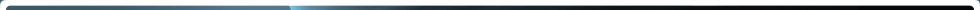There are four different but interrelated units for measuring radioactivity, exposure, absorbed dose, and dose equivalent. These can be remembered by the mnemonic R-E-A-D, as follows, with both common (British, e.g., Ci) and international (metric, e.g., Bq) units in use:

• Radioactivity refers to the amount of ionizing radiation released by a material. Whether it emits alpha or beta particles, gamma rays, x-rays, or neutrons, a quantity of radioactive material is expressed in terms of its radioactivity (or simply its activity), which represents how many atoms in the material decay in a given time period. The units of measure for radioactivity are the curie (Ci) and becquerel (Bq).

• Exposure describes the amount of radiation traveling through the air. Many radiation monitors measure exposure. The units for exposure are the roentgen (R) and coulomb/kilogram (C/kg).

• Absorbed dose describes the amount of radiation absorbed by an object or person (that is, the amount of energy that radioactive sources deposit in materials through which they pass). The units for absorbed dose are the radiation absorbed dose (rad) and gray (Gy).

• Dose equivalent (or effective dose) combines the amount of radiation absorbed and the medical effects of that type of radiation. For beta and gamma radiation, the dose equivalent is the same as the absorbed dose. By contrast, the dose equivalent is larger than the absorbed dose for alpha and neutron radiation, because these types of radiation are more damaging to the human body. Units for dose equivalent are the roentgen equivalent man (rem) and sievert (Sv), and biological dose equivalents are commonly measured in 1/1000th of a rem (known as a millirem or mrem).

For practical purposes, 1 R (exposure) = 1 rad (absorbed dose) = 1 rem or 1000 mrem (dose equivalent).

Note that a measure given in Ci tells the radioactivity of a substance, while a measure in rem (or mrem) tells the amount of energy that a radioactive source deposits in living tissue. For example, a person would receive a dose equivalent of 1 mrem from any one of the following activities:

• 3 days of living in Atlanta
• 2 days of living in Denver
• 1 year of watching television (on average)
• 1 year of wearing a watch with a luminous dial
• 1 coast-to-coast airline flight
• 1 year living next door to a normally operating nuclear power plant
Page Last Reviewed/Updated Monday, October 02, 2017

#### Home